# Python分析时间序列数据

### 范例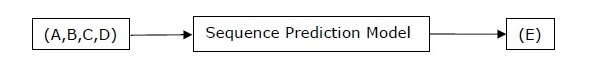Pandas是一个开源的BSD许可库，为Python提供高性能，易于使用的数据结构和数据分析工具。您可以借助以下命令安装Pandas：

pip install pandas


conda install -c anaconda pandas


### hmmlearn

pip install hmmlearn


### PyStruct

conda install -c omnia hmmlearn


### PyStruct

pip install pystruct


### CVXOPT

pip install cvxopt


conda install -c anaconda cvdoxt


### Pandas：从时间序列数据处理，切片和提取统计数据

• 使用pd.date_range包创建一系列日期
• 使用pd.Series包索引带有日期的pandas
• 使用ts.resample包执行重新采样
• 改变频率

### 处理时间序列数据

import numpy as np
import matplotlib.pyplot as plt
import pandas as pd


def read_data(input_file):
input_data = np.loadtxt(input_file, delimiter = None)


dates = pd.date_range('1950-01', periods = input_data.shape, freq = 'M')


output = pd.Series(input_data[:, index], index = dates)
return output

if __name__=='__main__':


input_file = "/Users/admin/AO.txt"


timeseries = read_data(input_file)


plt.figure()
timeseries.plot()
plt.show()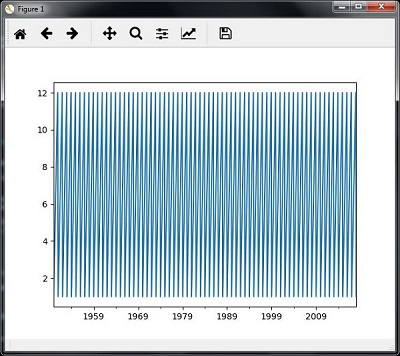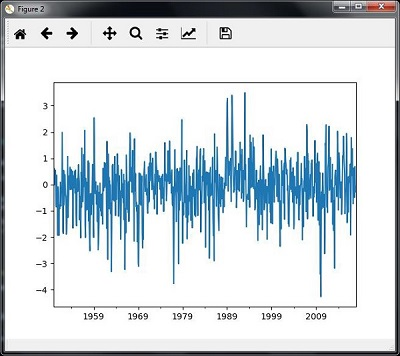### 切片时间序列数据

timeseries['1980':'1990'].plot()
<matplotlib.axes._subplots.AxesSubplot at 0xa0e4b00>

plt.show()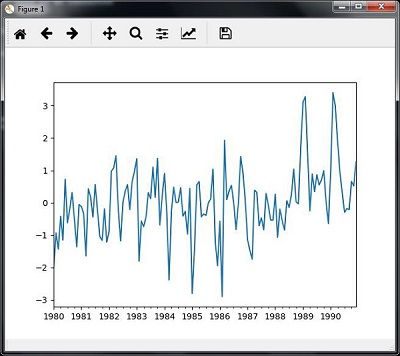### mean（）函数

timeseries.mean()


-0.11143128165238671


### max（）函数

timeseries.max()


3.4952999999999999


### min（）函数

timeseries.min()


-4.2656999999999998


### describe()

timeseries.describe()


count   817.000000
mean     -0.111431
std       1.003151
min      -4.265700
25%      -0.649430
50%      -0.042744
75%       0.475720
max       3.495300
dtype: float64


• 时间段
• 方法

### 用mean（）重新采样

timeseries_mm = timeseries.resample("A").mean()
timeseries_mm.plot(
plt.show()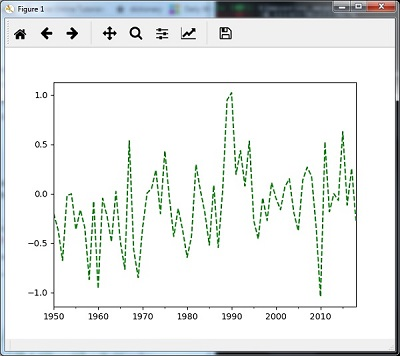### 用中位数重新取样（）

timeseries_mm = timeseries.resample("A").median()
timeseries_mm.plot()
plt.show()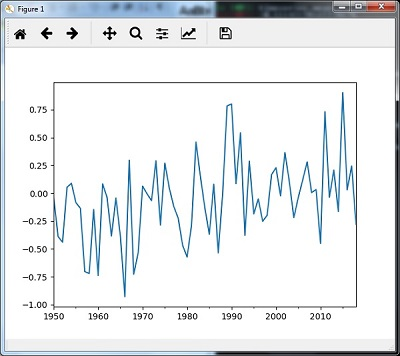### 滚动平均值

timeseries.rolling(window = 12, center = False).mean().plot(
plt.show()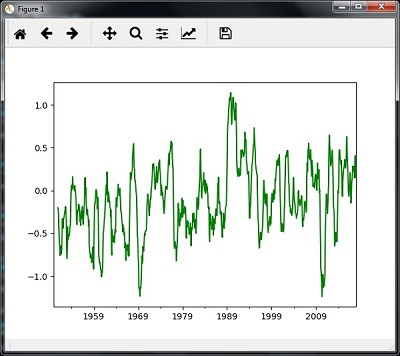### 先验概率矩阵（Π）

• S = {s1，s2，...，sN}是N个可能状态的集合，
• O = {o1，o2，...，oM}是一组M个可能的观察符号，
• A是NxN状态转换概率矩阵（TPM），
• B是NxM观测或发射概率矩阵（EPM），
• π是N维初始状态概率分布矢量。

### 示例：股市数据分析

import datetime
import warnings


import numpy as np
from matplotlib import cm, pyplot as plt
from matplotlib.dates import YearLocator, MonthLocator
try:
from matplotlib.finance import quotes_historical_yahoo_och1
except ImportError:
from matplotlib.finance import (
quotes_historical_yahoo as quotes_historical_yahoo_och1)

from hmmlearn.hmm import GaussianHMM


start_date = datetime.date(1995, 10, 10)
end_date = datetime.date(2015, 4, 25)
quotes = quotes_historical_yahoo_och1('INTC', start_date, end_date)


closing_quotes = np.array([quote for quote in quotes])


volumes = np.array([quote for quote in quotes])[1:]


diff_percentages = 100.0 * np.diff(closing_quotes) / closing_quotes[:-]
dates = np.array([quote for quote in quotes], dtype = np.int)[1:]
training_data = np.column_stack([diff_percentages, volumes])


hmm = GaussianHMM(n_components = 7, covariance_type = 'diag', n_iter = 1000)
with warnings.catch_warnings():
warnings.simplefilter('ignore')
hmm.fit(training_data)


num_samples = 300
samples, _ = hmm.sample(num_samples)


plt.figure()
plt.title('Difference percentages')
plt.plot(np.arange(num_samples), samples[:, 0], c = 'black')


plt.figure()
plt.title('Volume of shares')
plt.plot(np.arange(num_samples), samples[:, 1], c = 'black')
plt.ylim(ymin = 0)
plt.show()


Python语音识别：在本章中，我们将学习使用AI和Python进行语音识别。言语是成人人际交往的最基本手段。语音处理的基本目标是提供人与机器之间的交互。语音处理系统主要有三个任务 -首先 ，语音识别允许机器捕捉我们说的单词，短语和句子第二 ...